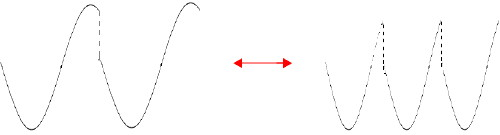RTcmix an open-source digital signal processing and sound synthesis language about · links · contact
SYNC -- 'hard' sync oscillator synthesis instrument
in RTcmix/insts/bgg

##### quick syntax:
SYNC(outsk, dur, PITCH, AMP, OSCFREQ, OSCWAVE[, PAN])

CAPITALIZED parameters are pfield-enabled for table or dynamic control (see the maketable or makeconnection scorefile commands). Parameters after the [bracket] are optional and default to 0 unless otherwise noted.

```   p0 = output start time (seconds)
p1 = duration (seconds)
p2 = pitch (Hz or oct.pc)
p3 = amplitude (absolute, for 16-bit soundfiles: 0-32768)
p4 = oscillator writing frequency
p5 = oscillator wavetable reference
p6 = pan [optional; default is 0]

p2 (pitch), p3 (amplitude), p4 (oscillator writing frequency) and p6 (pan)

p5 (oscillator wavetable reference) should be a reference to a pfield table-handle.

```

SYNC periodically resets the writing of an oscillating waveform (i.e. resets the writing phase to 0), 'hard' syncing the writing oscillator to the reset rate.

### Usage Notes

The rate at which the 'writing' oscillator gets periodically reset to phase 0 determines the pitch of resulting output. This rate is thus determined by parameter p2 ("PITCH"). p2 can be either oct.pc or Hz (< 15.0 is the switch-over from Hz to oct.pc).

By modulating the frequency of the 'writing' oscillator (p4, "OSCFREQ"), dynamically changing timbral effects are possible because the waveform being written between resets of the oscillator will shift:Any arbitrary wavetable can be used by the 'writing' oscillator. The waveform is specified through the pfield-table reference in p5 ("OSCWAVE").

SYNC can produce both mono and stereo output.

### Sample Scores

very basic:
```   rtsetparams(44100, 1)

ampenv = maketable("line", 1000, 0,0, 1,1, 9,1, 10,0)

wave = maketable("wave", 1000, "sine")
wdex = maketable("line", "nonorm", 1000, 0, 200, 1, 400, 2, 200)

SYNC(0, 3.5, 225, 20000*ampenv, wdex, wave)
```

```   rtsetparams(44100, 2)

amp = 8000
ampenv = maketable("line", 1000, 0,0, 1,1, 9,1, 10,0)

wave = maketable("wave", 1000, "saw7")

for (i = 0; i < 7; i += 1) {
pchenv = maketable("line", "nonorm", 1000, 0, irand(5.065, 5.075),
irand(1, 5), irand(5.065, 5.075),
irand(5, 9), irand(5.065, 5.075), 10, 5.07)

wdex = maketable("line", "nonorm", 1000, 0, irand(100, 500), irand(1, 3),
irand(100, 500), irand(4, 7),
irand(100, 500), irand(8, 10), irand(100, 500))

SYNC(0, 14, pchenv, amp*ampenv, wdex, wave, random())
}
```

fun stuff!
```   rtsetparams(44100, 2)

reset(10000)

pches = { 0.00, 0.01, 0.03, 0.04, 0.06, 0.09, 0.12 }
lpches = len(pches)
bpitch = 7.09

amp = 10000
ampenv = maketable("line", 1000, 0,0, 1,1, 9,1, 10,0)

wave = maketable("wave", 1000, "saw3")
wdex = maketable("line", "nonorm", 1000, 0, 400, 1, 200)

st = 0
dur = 0.15
for (i = 0; i < 78; i += 1) {
pch = bpitch + pches[trand(0, lpches)]
SYNC(st, dur, pch, amp*ampenv, wdex, wave, random())
st += irand(0, dur)
}

```